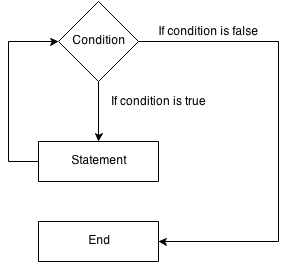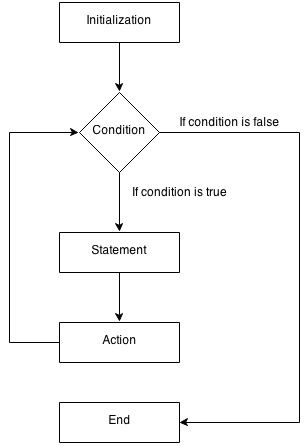### Loop and Iteration

A loop statement allows us to iterate a/a group of statement multiple times.

You can write a loop statement using for or while :

### While loop

``` while ( condition ) {    continue execute this statement if the condition is true } ```### For loop

``` for ( initialization; condition ; action after each iteration ) {    continue execute this statement if the condition is true } ```### Practice

using namespace std ;

for( int counter = 1; counter <= 10; counter++)

{

cout<<"Value of "<< counter << " x 4 is " << counter*4 << endl;

}

### Output

//When counter =
Value of 1 x 4 is 4

//Whole output
Value of 1 x 4 is 4
Value of 2 x 4 is 8
Value of 3 x 4 is 12
Value of 4 x 4 is 16
Value of 5 x 4 is 20
Value of 6 x 4 is 24
Value of 7 x 4 is 28
Value of 8 x 4 is 32
Value of 9 x 4 is 36
Value of 10 x 4 is 40